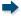Pre-Calculus - Maplesoft Books - Maple Books, Maple Resources and Math BooksHome : Maplesoft Books : Pre-CalculusPre-Calculus Search
 Trigonometric Equations - The Told and Untold Raymond Sarkissian 2016 Pre-Calculus
 Sigurd Eriksson Matematik DEL II Sigurd Eriksson 2015 Pre-Calculus
 Sigurd Eriksson Matematik DEL I Lennart Gombrii 2013 Pre-Calculus
 Maple 15 Programming Guide Maplesoft 2011 Calculus | Computer Algebra | Differential Equations | Pre-Calculus
 Discovering Mathematics: A Problem-Solving Approach to Mathematical Analysis with MATHEMATICA® and Maple(TM) Jirí Gregor, Jaroslav Tišer 2010 Calculus | Complex Analysis | Differential Equations | Engineering | Numerical Analysis | Pre-Calculus
 Getting Started with Maple, 3rd ed. Douglas B. Meade, Mike May, S.J., C-K. Cheung, G.E. Keough 2009 Calculus | Computer Algebra | Differential Equations | Linear Algebra | Pre-Calculus | Programming | Vector Calculus
 Laboratorio de Matemáticas (Tomo I): Números y Ecuaciones L. González-Vega 2007 Number Theory | Pre-Calculus
 14 colles en Maple J. C. Leicknam 2001 Pre-Calculus | Calculus
 Analytical Geometry I. Vaisman 1998 Calculus | Pre-Calculus
 1 2Page 1 of 2
 Do you know of a book that should be included in our list? Tell us about it!
 Title/Author: Category: Any Category Abstract Algebra Biology Calculus Chemistry Complex Analysis Computer Algebra Computer Science Conference Proceedings Courseware Cryptography Differential Equations Differential Geometry Dynamical Systems Economics Engineering Games Linear Algebra Maple Manuals Mathematical Modeling Number Theory Numerical Analysis Operations Research Optimization Physics Pre-Calculus Probability & Statistics Programming Recent Releases Vector Calculus Language: Any Language English French German Spanish Japanese Dutch Russian Swedish Polish Persian ChineseAbstract Algebra Biology Calculus Chemistry Complex Analysis Computer Algebra Computer Science Conference Proceedings Courseware Cryptography Differential Equations Differential Geometry Dynamical Systems Economics Engineering Games Linear Algebra Maple Manuals Mathematical Modeling Number Theory Numerical Analysis Operations Research Optimization Physics Pre-Calculus Probability & Statistics Programming Recent Releases Vector Calculus